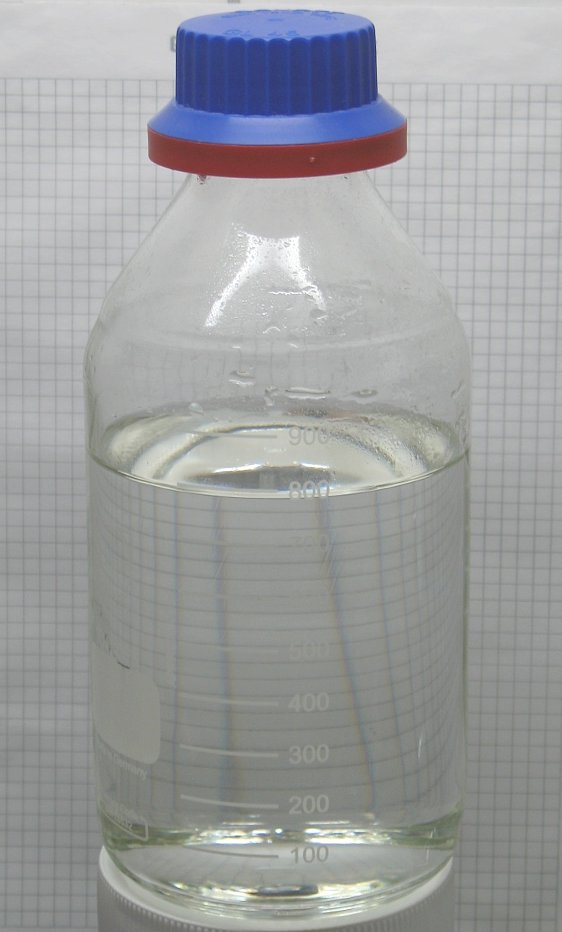## Binary Acids

#### Learning Objective

• Explain the periodic trends that affect binary acid strength.

#### Key Points

• A binary acid’s strength depends on the H-X bond’s strength; the weaker the bond, the stronger the acid.
• The names of binary acids begin with “hydro-” followed by the name of the other element, modified to end with “-ic.”
• Binary acids are one of two classes of acids; the second are oxoacids (or oxyacids), which consist of hydrogen, oxygen, and another element.

#### Terms

• binary acidmolecular compounds in which hydrogen is combined with a second nonmetallic element
• pKaa quantitative measure of the strength of an acid in solution; a weak acid has a pKa value in the approximate range −2 to 12 in water, and a strong acid has a pKa value of less than about −2.

## Acid Strength and Bond Strength

Binary acids are certain molecular compounds in which hydrogen is combined with a second nonmetallic element; these acids include HF, HCl, HBr, and HI.

HCl, HBr, and HI are all strong acids, whereas HF is a weak acid. The acid strength increases as the experimental pKa values decrease in the following order:

HF (pKa = 3.1) < HCl (pKa = -6.0) < HBr (pKa = -9.0) < HI (pKa = -9.5).

Why is HF a weak acid, when the rest of the hydrohalic acids are strong? One might correctly assume that fluorine is very electronegative, so the H-F bond is highly polar and we can expect HF to dissociate readily in solution; this reasoning is not wrong, but the electronegativity argument is trumped by considerations of ionic size. Recall the periodic trend that ionic size increases as we move down the periodic table. Because fluorine is at the top of the halogens, the Fion is the smallest halide; therefore, its electrons are concentrated around its nucleus, and as a result, the H-F bond is relatively short. Shorter bonds are more stable, and thus the H-F bond is more difficult to break.

Once we move down to chlorine, howver, the trend changes. Chlorine is larger and has more electrons, and therefore the H-Cl bond is longer and weaker. In the presence of water, the electrostatic attractions between water’s partially negative oxygen and the partially positive hydrogen on H-Cl is strong enough to break the H-Cl bond, and the ions dissociate in solution.

The same reasoning applies for both HBr and HI. These acids are even stronger than HCl because the Br and I ions are even larger. As such, the H-Br and H-I bonds are even weaker, and these compounds also readily dissociate in solution.

## Naming Binary Acids

The names of binary acids begin with “hydro-” followed by the name of the other element, modified to end with “-ic.” For example, HCl is named hydrochloric acid.

Binary acids are one of two classes of acids, the second being oxoacids (or oxyacids), which consist of hydrogen, oxygen, and a third element, which is often a non-metal.Hydrochloric acidHydrochloric acid is a clear, colorless solution of hydrogen chloride (HCl) in water. It is a highly corrosive, strong mineral acid with many industrial uses. Hydrochloric acid is found naturally in gastric acid. It is a member of the binary acids.